# Electric Fields and Pollution Electric Fields Coulombs Law

• Slides: 15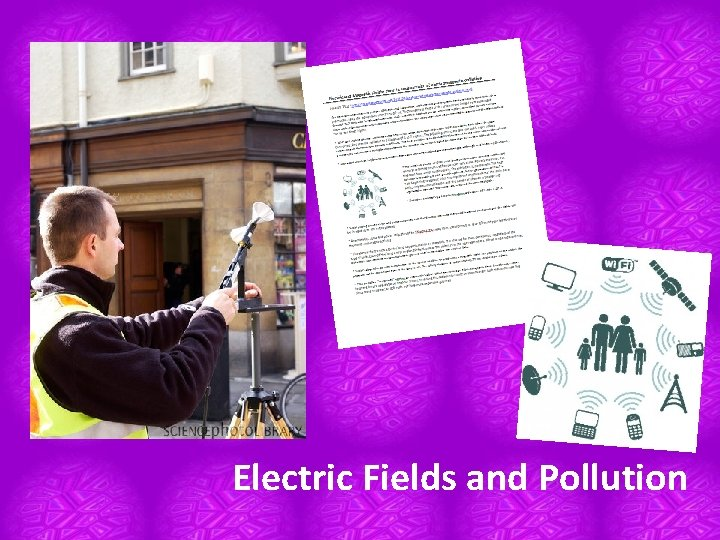Electric Fields and Pollution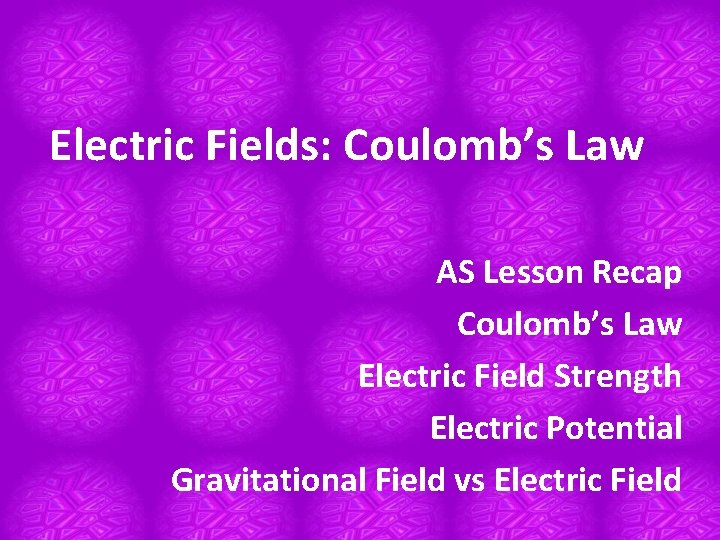Electric Fields: Coulomb’s Law AS Lesson Recap Coulomb’s Law Electric Field Strength Electric Potential Gravitational Field vs Electric FieldRecap • An electric field is a field of force that can be represented by field lines.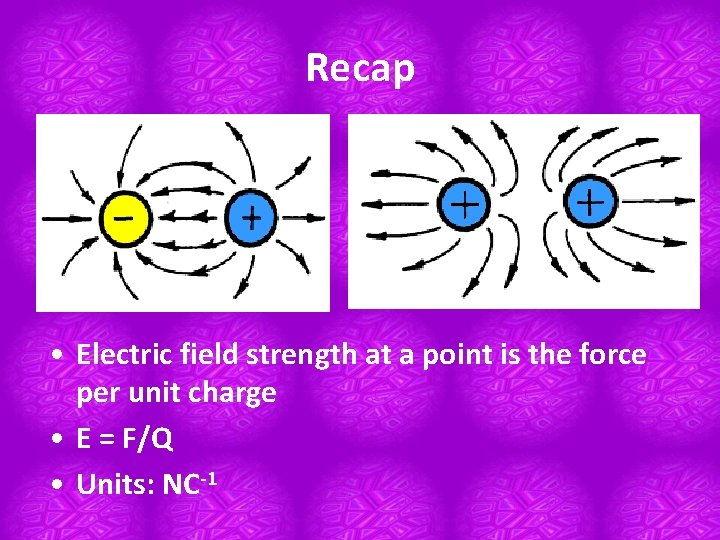Recap • Electric field strength at a point is the force per unit charge • E = F/Q • Units: NC-1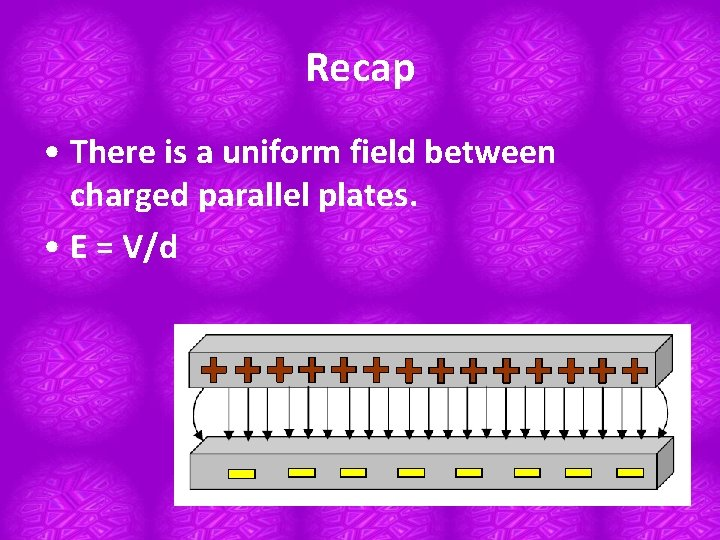Recap • There is a uniform field between charged parallel plates. • E = V/dCoulomb’s Law Torsional Balance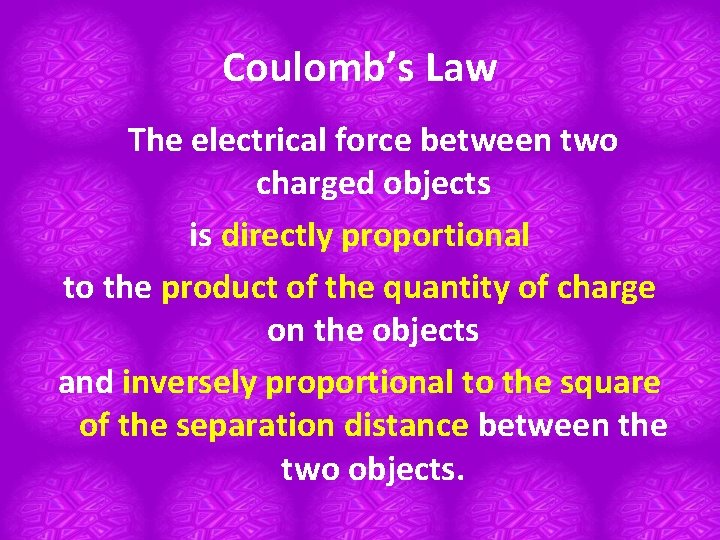Coulomb’s Law The electrical force between two charged objects is directly proportional to the product of the quantity of charge on the objects and inversely proportional to the square of the separation distance between the two objects.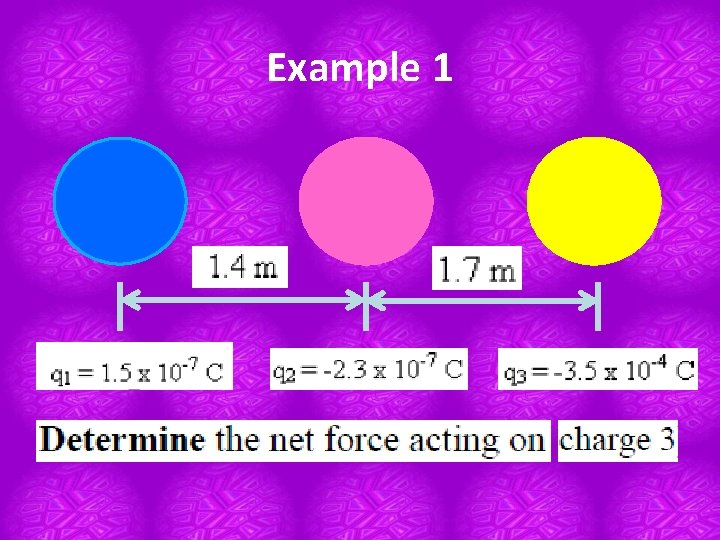Example 1Example 2 Two 1. 1 -gram balloons are suspended from 2. 0 -meter long strings and hung from the ceiling. They are then rubbed ten times with animal fur to impart an identical charge Q to each balloon. The balloons repel each other and each string is observed to make an angle of 15 degrees with the vertical. Determine the electric force of repulsion, the charge on each balloon (assumed to be identical), and the quantity of electrons transferred to each balloon as a result of 10 rubs with animal fur.Example 3 Three charges are placed along the x-axis. Charge A is a +18 n. C charge placed at the origin. Charge B is a -27 n. C charge placed at the 60 cm location. Where along the axis must positively charged C be placed in order to be at equilibrium?Electric Field Strength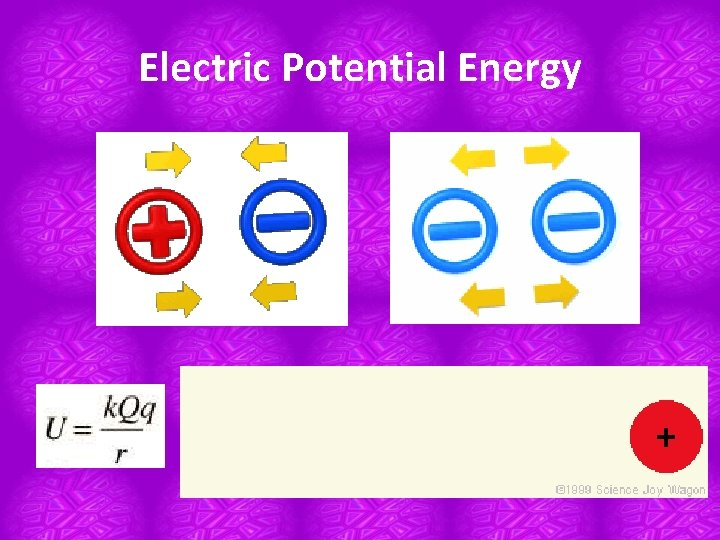Electric Potential Energy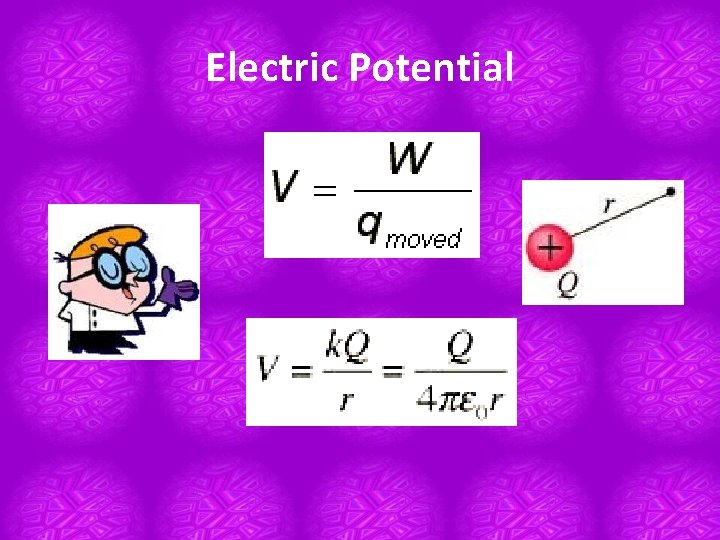Electric PotentialElectric Potential at a point Work done in bringing unit positive charge from infinity to the pointGravitational field vs Electric field